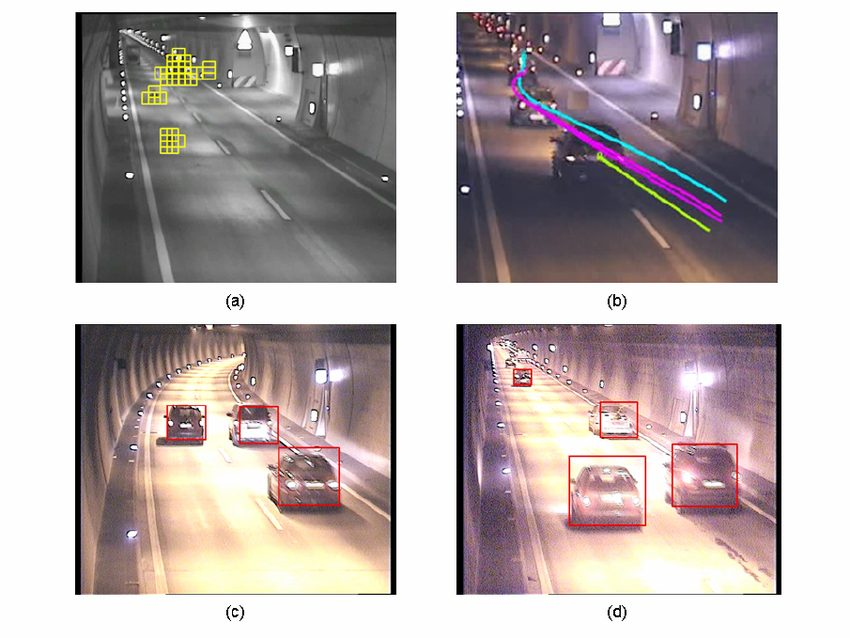## Object Location and Tracking In Image Processing

Dividing and organizing an image in the desired way and detecting and isolating the edges of desired objects are the key components in recognizing and then tracking the objects. There are countless algorithms already in use for detecting and tracking objects, including people. Which application and outcome is targeted determines which one or which combination of multiple is used. Many algorithms have a basis using a Bayesian approach. Bayes theorem is a statistical theorem that relates current probability to prior probability. Particle filters are density estimator algorithms that use Bayesian recursion to estimate the probability of hidden parameters based on observable data. These filters are known to be effective and are frequently used in object tracking.
An often explored algorithm used both by itself and in conjunction with other approaches is the Iterative Closest Point Algorithm (ICP). This algorithm relates corresponding sets of points and attempts to minimize the difference between them by iteratively translating and rotating points until a solution converges. Aligning the point sets allows for efficient feature recognition used in object recognition and motion tracking. Although this algorithm is efficient in both two and three dimensional applications, often times it is unable to effectively function when applied to rapidly moving human body motion. Various modifications have been applied to solve this problem including fitting the ICP algorithm with a modified particle filter algorithm discussed previously. Template matching is another common method used to identify objects and object positions in a noisy environment. A template, either binary, color, or greyscale, translates over the target image, and at each place, a comparison is made to determine whether the template matches the object. Template matching can be an effective and efficient method when it is known that a specific object exists in the frame, and an estimate of the pattern is known. Medical image processing, facial and body part recognition, and traffic feature detection are just a few common applications.
Cross correlation, also known as the sliding dot product, measures the similarity between two inputs. In image processing, the inputs are usually a target image and a template that is to be matched to this image. Geometrically, the dot product can be defined as the product of the Euclidean norm of the two vectors and the cosine of the angle between them. Within template matching, this means that in order to have a maximum similarity between the template and the target image, the cosine of the angle needs to be at a minimum to therefore maximize the dot product. Cross correlation is called a sliding dot product as the dot product is continuously calculated as the template translates across the target image in discrete steps until an acceptable correlation value is calculated. This value is typically normalized, as normalized cross correlation values allow for better comparison. As this can be a tedious process, especially if no known approximation of where the target object is located exists, modifications are typically made for a more efficient algorithm. Creating pre-calculated sum tables and approximating the template function aids in more efficient calculations.
Chamfer matching is, at the moment, the most efficient method of shape matching. This algorithm reduces the number of edges to match and instead uses the minimum number of defined and unvarying edges to yield quick and accurate matches. This is especially useful when analyzing low level images. Some have improved the basic form of this algorithm by incorporating edge orientation which creates a smoother loss function. This method can be further simplified while still maintaining an acceptable level of accuracy by incorporating a theory based on centroidal Voronoi tessellations to use fewer pixels. Reducing the number of points used is accomplished in two ways. One of these is by adjusting the point densities of both the target image and the template. The other is optimizing the measured distances between points so that removing the points keeps the distance map the same.
To further increase the efficiency of template matching, a hierarchy of templates can be created. Considering how computationally expensive it is to compare a single template over an entire image using discrete steps, even if using the Fast Normalized Cross Correlation method described above, improvements are always sought to improve its effectiveness. Rather than searching through every template one by one, and possibly missing matches due to slight scaling and resolution differences, a hierarchy allows the templates to be grouped so that an initial general template grouped is first determined and
then sorted like so through the lower levels of that group. Because objects in a scene can be depicted at different scales and resolutions, having a pyramid of templates to sort through significantly reduces computational time.Object Location and Tracking In Image Processing